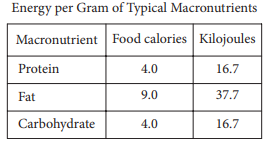mycollegehive
The table above gives the typical amounts of energy per gr...
SAT-math-test SAT math0The table above gives the typical amounts of energy per gram, expressed in both food calories and kilojoules, of the three macronutrients in food.

If the 180 food calories in a granola bar come entirely from p grams of protein, f grams of fat, and c grams of carbohydrate, which of the following expresses f in terms of p and c ?

A) f = 20 + 4/9 (p + c)

B) f = 20 - 4/9 (p + c)

C) f = 20 - 4/9 (p - c)

D) f = 20 + 9/4 (p + c)

1.2K viewsShareFollowAsked by judziyrep:1.9K
University of Benin Nigeria
30 May 2020

Comments

### 1 answer(s)

Advertisement (continue below)Peter answeredrep:1.7K
University of Lagos Nigeria
18 August 20200There are 4 food calories per gram of protein, 9 food calories per gram of fat and 4 food calories per gram of carbohydrate

If 180 food calories in a granola bar comes entirely from p grams of protein, f grams of fat, and c grams of carbohydrate,

180 = 4p + 9f + 4c

9f = 180 - 4p - 4c

9f = 180 - 4(p + c)

divide through by 9

f = 20 - 4/9 (p + c)

Ans BShare
Comments

Advertisement (continue below)

### Groups

Advertisement (continue below)

Your Answer

How to insert math formulas/equations### Related Tags

SAT-math-test

13 followers

476 questionsSAT

7 followers

487 questionsmath

20 followers

1261 questions